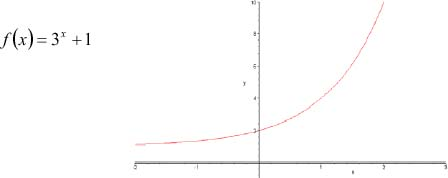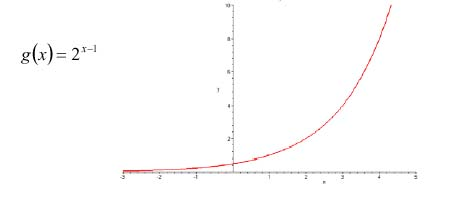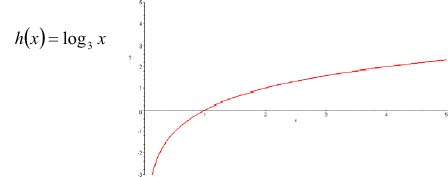Graphing Functions and Logarithms

Graphing Functions and Logarithms

Practice

1.    Graph f(x) = 3x + 1

2.    Graph  g(x) = 2x - 1

3.  Graph  h(x) = log3x

1.2.3.